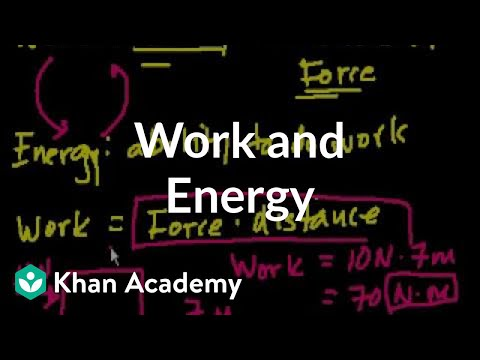### Main Difference

The work and energy are closely associated terms in physics due to which it sometimes get hard to differentiate between them. The work is the process in which energy transfer takes place, and the objects travel in the direction of the force applied. On the other hand, energy is the ability of the body through which one can do the work. Moreover, the energy can neither be created nor be destroyed.

### Comparison Chart

 Work Energy Definition The force applied to the object makes it move in the direction of the force. The ability to produce or create work. Name Source In the field of physics it was introduced by the French mathematician Gaspard-Gustave Coriolis back in 1826. Aristotle used this term back in 4th century BC. Derived from Greek work ‘energia’. Types No Electrical energy, chemical energy, heat, nuclear energy and many other.

### What is Work?

The work is the compact process in the field of physics under which the energy conversion takes place, and the energy applied to the object leads the work done after it travels in the direction of the energy applied. In a layman’s dictionary, the chores like reading and thinking are even the work, but this doesn’t stand stable on the work’s definition provided by the physics. The work done is the product of the force applied to the object and the distance it has covered in the direction of the force. It will be compulsory to mention here that if the energy conversion is taking place or the force is applied to the object, and still no distance is covered by the object then no work is done. Force (converted energy) and distance traveled in the direction of the force are the necessary elements of the work done. At the same time, the concept of energy conversion stands along with it as the energy conversion works as the required force on the object. The work is a scalar quantity that means it doesn’t require direction for its representation. In other words, we can say that the final product we get by multiplying the force applied and the distance traveled is the joules, and it has nothing to with the concept of the direction requirement for the representation. The term ‘work’ in the field of physics was introduced by the French mathematician Gaspard-Gustave Coriolis back in 1826.

### Example of Work

Work Done

Our walking is one of the simplest examples of the work done, where the stored energy (mechanical energy) gets converted into the kinetic energy and makes it resistance to the ground, which results in pushing ourselves forward and leaving the ground behind. In this process, the certain force by the body is applied and the traveling of the body in the direction of the force is also seen.

Work Not Done

If we spend our time pushing the wall and it doesn’t move a single inch then it means no work is done regardless the mighty force applied on it. Hence, the concept of the distance covered in the direction of the force is one of the main essences of the work done.

### What is Energy?

Energy is the ability to work; it comes in the dozens of forms and generally whenever the work is done an energy conversion is a compulsory act of that time. The term energy is closely associated with the concept of the work done as it works as the force in work, which is a necessary element in work. The energy can neither be created and nor be destroyed it keeps on changing from one form to the other. The conversion of the energy leads to the process of work done; especially the kinetic energy which lets the object travel in the specific direction. As we know that energy comes in different forms namely electrical energy, chemical energy, heat, nuclear energy; mainly all these are classified either as the potential and kinetic energy or the renewable or non-renewable energy (by the energy sources). Like work, energy is also the scalar quantity and has Joule as its SI unit. The term energy is said to be derived from the Greek word ‘energia,’ which means activity or operation. Aristotle is recognized as the one introducing this term back in the 4th century BC.

### Example of Energy

For instance, we took the meal, which comprises of the chemical energy. Further, the chemical energy gets stored in the form of potential energy and when this energy is capitalized the potential energy gets converted into the kinetic energy.

### Work vs. Energy

• Work is the distance traveled by the object in the direction of the force applied, whereas the energy acts as the force and it refers the ability of the object to produce or create work.
• The energy is the older term as Aristotle introduced it in 4th century BC. Contrary to this, the work is the modern term in the physics, which was introduced in 1826 by the French mathematician.
• Work has no types; it is the product of the force and the distance covered by the object. Conversely, energy has dozens of types; out of them, some are heat, light, nuclear energy, electrical energy and the chemical energy.
• The process of work done takes place after the energy is transferred. The potential energy is transferred into the kinetic energy for the work to be done.